#### Defining new neighborhoods

For defining new neighborhoods, it is important to keep them simple because during execution, the neighborhoods that contain the statemust be determined quickly. Suppose that all neighborhoods are open balls: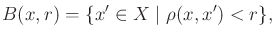(8.52)

in which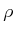is the metric on. There are efficient algorithms for determining whether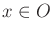for some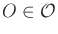, assuming all of the neighborhoods are balls . In practice, methods based on Kd-trees yield good performance [47,52] (recall Section 5.5.2). A new ball,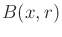, can be constructed in Step 3 for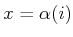, but what radius can be assigned? For a point robot that translates in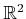or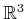, the Hausdorff distance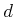between the robot and obstacles in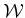is precisely the distance to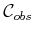from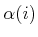. This implies that we can set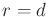, andis guaranteed to be collision-free.

In a general configuration space, it is possible to find a value of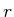such that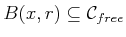, but in general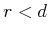. This issue arose in Section 5.3.4 for checking path segments. The transformations of Sections 3.2 and 3.3 become important in the determination of. For illustrative purposes, suppose that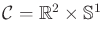, which corresponds to a rigid robot,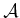, that can translate and rotate in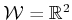. Each point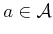is transformed using (3.35). Now imagine starting with some configuration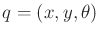and perturbing each coordinate by some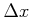,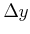, and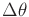. What is the maximum distance that a point oncould travel? Translation affects all points onthe same way, but rotation affects points differently. Recall Figure 5.12 from Section 5.3.4. Let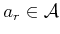denote the point that is furthest from the origin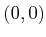. Letdenote the distance from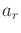to the origin. If the rotation is perturbed by some small amount,, then the displacement of anyis no more than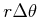. If all three configuration parameters are perturbed, then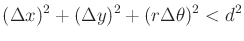(8.53)

is the constraint that must be satisfied to ensure that the resulting ball is contained in. This is actually the equation of a solid ellipsoid, which becomes a ball if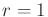. This can be made into a ball by reparameterizing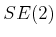so thathas the same affect asand. A transformation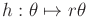maps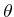into a new domain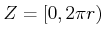. In this new space, the equation of the ball is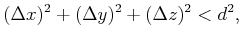(8.54)

in which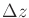represents the change in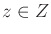. The reparameterized version of (3.35) is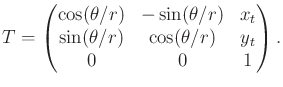(8.55)

For a 3D rigid body, similar reparameterizations can be made to Euler angles or quaternions to generate six-dimensional balls. Extensions can be made to chains of bodies . One of the main difficulties, however, is that the balls are not the largest possible. In higher dimensions the problem becomes worse because numerous balls are needed, and the radii constructed as described above tend to be much smaller than what is possible. The number of balls can be reduced by also allowing axis-aligned cylinders, but it still remains difficult to construct a cover over a large fraction ofin more than six dimensions.

Steven M LaValle 2020-08-14